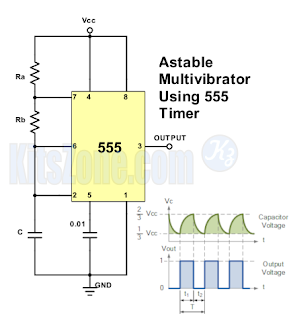The IC 555 timer is connected for astable operation. Only one resistor is used as timing resistor in monostable multivibrator using 555 and here the timing resistor is split into two sections as Ra and Rb, with the discharge pin (pin 7) is connected to the junction of Ra and Rb.

The IC 555 timer is connected for astable operation. Only one resistor is used as timing resistor in monostable multivibrator using 555 and here the timing resistor is split into two sections as Ra and Rb, with the discharge pin (pin 7) is connected to the junction of Ra and Rb. When the power supply is switched on, the timing capacitor C charges towards 2/3Vcc through Ra and Rb. When the capacitor voltage reaches 2/3 of Vcc, the upper comparator triggers the flip-flop and the capacitor starts to discharge towards ground through Rb. When the discharge reaches 1/3 of Vcc, the lower comparator is triggered and a new cycle is started.
The capacitor is charged and discharged periodically between 1/3 of Vcc and 2/3 of Vcc.
The output stage is High during the charging cycle for a time period t1, so

$$t_{1}=(R_{a}+R_{b})C \text{ } ln{\frac{V_{cc}-\frac{1}{3}V_{cc}}{V_{cc}-\frac{2}{3}V_{cc}}}$$
$$t_{1}=(R_{a}+R_{b})C \text{ } ln{\frac{3V_{cc}-V_{cc}}{3V_{cc}-{2}V_{cc}}}$$
$$t_{1}=(R_{a}+R_{b})C \text{ } ln{\frac{2V_{cc}}{V_{cc}}}$$
$$t_{1}=(R_{a}+R_{b})C \text{ } ln{2}$$
$$\therefore t_{1}=0.693(R_{a}+R_{b})C ---- (1)$$

The output stage is low during the discharge cycle for a time period t2
$$\therefore t_{2}=0.693(R_{b})C ---- (2)$$

So, the total time period for charging and discharging is,
$$T=t_{1}+t_{2}$$
$$T=0.693(R_{a}+2R_{b})C$$

Output frequency is given as,
$$f=\frac{1}{T}=\frac{1.443}{(R_{a}+2R_{b})C}$$

DUTY CYCLE
The duty cycle D of a recurring output is defined as the ratio of the High time to the total cycle,
$$D=\frac{t_{1}}{T}=\frac{R_{a}+R_{b}}{R_{a}+2R_{b}}$$Amazon Affiliate Disclaimer:The owner of this website(ecircuits.tk) is a participant in the Amazon India Associates Programme, an affiliate advertising programme designed to provide a means for sites to earn advertising fees by advertising and linking to Amazon.in.
Name

Alarm circuit,2,Audio Amplifier Circuit,1,Audio Circuit,2,charger circuit,3,Controller circuit,1,Emergency Light Circuit,2,Indicator Circuit,3,Inverter Circuit,1,LED Circuit,4,Multivibrator Circuits,1,Power supply,1,Rectifier Circuit,1,
ltr
item
Simple Electronic Circuits: Astable Multivibrator Using IC 555 Tmer Circuit Diagram
Astable Multivibrator Using IC 555 Tmer Circuit Diagram
The IC 555 timer is connected for astable operation. Only one resistor is used as timing resistor in monostable multivibrator using 555 and here the timing resistor is split into two sections as Ra and Rb, with the discharge pin (pin 7) is connected to the junction of Ra and Rb.
https://1.bp.blogspot.com/-RCqfgO39Gfo/W_tlQuSV4_I/AAAAAAAABCE/p3I_lgkBMu8RDdiVylOiLnHQ9lfhHPxVQCKgBGAs/s320/Astable-multi-using-555.png
https://1.bp.blogspot.com/-RCqfgO39Gfo/W_tlQuSV4_I/AAAAAAAABCE/p3I_lgkBMu8RDdiVylOiLnHQ9lfhHPxVQCKgBGAs/s72-c/Astable-multi-using-555.png
Simple Electronic Circuits
https://www.ecircuits.tk/2018/11/astable-multivibrator-using-ic-555-tmer.html
https://www.ecircuits.tk/
https://www.ecircuits.tk/
https://www.ecircuits.tk/2018/11/astable-multivibrator-using-ic-555-tmer.html
true
4078171261878103471
UTF-8